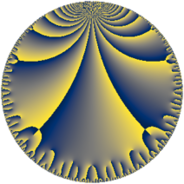# Properties

 Label 38.10.eLevel $38$ Weight $10$ Character orbit 38.e Rep. character $\chi_{38}(5,\cdot)$ Character field $\Q(\zeta_{9})$ Dimension $90$ Newform subspaces $2$ Sturm bound $50$ Trace bound $1$

# Related objects

## Defining parameters

 Level: $$N$$ $$=$$ $$38 = 2 \cdot 19$$ Weight: $$k$$ $$=$$ $$10$$ Character orbit: $$[\chi]$$ $$=$$ 38.e (of order $$9$$ and degree $$6$$) Character conductor: $$\operatorname{cond}(\chi)$$ $$=$$ $$19$$ Character field: $$\Q(\zeta_{9})$$ Newform subspaces: $$2$$ Sturm bound: $$50$$ Trace bound: $$1$$ Distinguishing $$T_p$$: $$3$$

## Dimensions

The following table gives the dimensions of various subspaces of $$M_{10}(38, [\chi])$$.

Total New Old
Modular forms 282 90 192
Cusp forms 258 90 168
Eisenstein series 24 0 24

## Trace form

 $$90q - 219q^{3} + 4560q^{6} - 11436q^{7} - 12288q^{8} + 20805q^{9} + O(q^{10})$$ $$90q - 219q^{3} + 4560q^{6} - 11436q^{7} - 12288q^{8} + 20805q^{9} - 22596q^{11} - 124416q^{12} + 373014q^{13} + 193728q^{14} - 1114176q^{15} + 2130090q^{17} + 2718624q^{18} + 3104982q^{19} - 872448q^{20} - 8253738q^{21} - 3975936q^{22} - 5339532q^{23} + 1167360q^{24} + 18934260q^{25} - 1147296q^{26} - 9159261q^{27} + 1488384q^{28} + 18350388q^{29} + 2615190q^{31} - 18138567q^{33} - 319680q^{34} - 4456146q^{35} + 5326080q^{36} + 69738420q^{37} - 40163472q^{38} - 73267932q^{39} - 33950949q^{41} + 15660864q^{42} + 140463276q^{43} - 16809984q^{44} + 42856866q^{45} - 23232000q^{46} + 280493580q^{47} + 28704768q^{48} - 142532535q^{49} - 246449616q^{50} - 256360335q^{51} - 141161472q^{52} - 244750782q^{53} + 5545872q^{54} + 493500528q^{55} + 329170944q^{56} + 512355660q^{57} + 103047552q^{58} - 247376757q^{59} - 166388736q^{60} - 717568098q^{61} - 376369632q^{62} - 225437622q^{63} - 754974720q^{64} + 76903230q^{65} + 930585648q^{66} + 1376150385q^{67} + 278354688q^{68} - 696062304q^{69} - 1447925952q^{70} - 1744555950q^{71} + 40132608q^{72} + 1380900810q^{73} + 309873888q^{74} + 2657812500q^{75} - 235451904q^{76} + 426872076q^{77} - 1076816928q^{78} - 2723006472q^{79} + 2045417583q^{81} + 2465868336q^{82} + 1266060108q^{83} - 102749184q^{84} - 498757524q^{85} - 365688384q^{86} - 1158620358q^{87} - 359817216q^{88} - 1215699516q^{89} - 2740985664q^{90} - 2339391108q^{91} - 171485184q^{92} + 9035460222q^{93} + 5158671168q^{94} - 1090068390q^{95} - 3216818607q^{97} + 1403099136q^{98} - 5695561353q^{99} + O(q^{100})$$

## Decomposition of $$S_{10}^{\mathrm{new}}(38, [\chi])$$ into newform subspaces

Label Dim. $$A$$ Field CM Traces $q$-expansion
$$a_2$$ $$a_3$$ $$a_5$$ $$a_7$$
38.10.e.a $$42$$ $$19.571$$ None $$0$$ $$-252$$ $$0$$ $$14373$$
38.10.e.b $$48$$ $$19.571$$ None $$0$$ $$33$$ $$0$$ $$-25809$$

## Decomposition of $$S_{10}^{\mathrm{old}}(38, [\chi])$$ into lower level spaces

$$S_{10}^{\mathrm{old}}(38, [\chi]) \cong$$ $$S_{10}^{\mathrm{new}}(19, [\chi])$$$$^{\oplus 2}$$